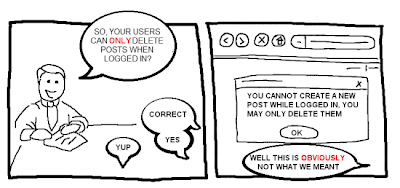## >>  Thursday, January 12, 2012

Thomas Kyte (a god in my work world and a pin-up in my office) questioned this question.  Anybody that is familiar with Tom knows that he tends to question any question.  There is a problem in general that questions tend to be remarkably ambiguous and often the answer is "it depends".

If you choose an answer to this question at random, what is the chance you will be correct?
a) 25%
b) 50%
c) 60%
d) 25%
Seems really simple at first glance doesn't it? 50%: Now think about it.

I'm going to quote Tom word for word and hope that he forgives me if I tell you to buy one of his books.  If you are an Oracle DBA and don't own a TK book you should be ashamed of yourself.

"They are missing the correct answer in the list!  And adding the correct answer would change the answer - so that it might be incorrect as well.  Meaning - there is more missing here than supplied.  You don't stand a chance of answering the question at all.

I put forth that the answer is "e) none of the above" - making the right answer "20%".

Looking at the problem, if you assume the right answer is 25% - because you have a 1 in 4 chance of selecting any one of the items - the probability you will get the right answer randomly is 50%.  So, it cannot be 25% - if it were - it would be 50% (confusing, yes).

Moreover, if you assume 50% is correct, then the right answer is 25% - because the chance of picking 50% is 1 in 4.

If you assume 60% is correct - well, I don't know what to say.

The question itself seems to somehow affect the answer - the act of answering the question changes the answer.  Sort of like when you run a program in the debugger and it works perfectly - but when you run it standalone it crashes (that has never happened to me, nope, not a chance...).So, my answer is "e) none of the above"

The problem can be pushed further is you replace the 60% with 0%.  If 0% was correct, the odds would be 25%, but there are 2 25%'s so it would be 50%, but there is only 1 50% so it would be 25% and so on. Infinite loop.

The original question was posted here, the comments section is worth a read.  Nearly every time I read a different answer I agree with it.Source

The problem really is with the question.  Knowing what you are asking is in someways more important than the answer.  Which leads us right into the Ultimate Question.  The answer to which we know but the question has never been quite understood.